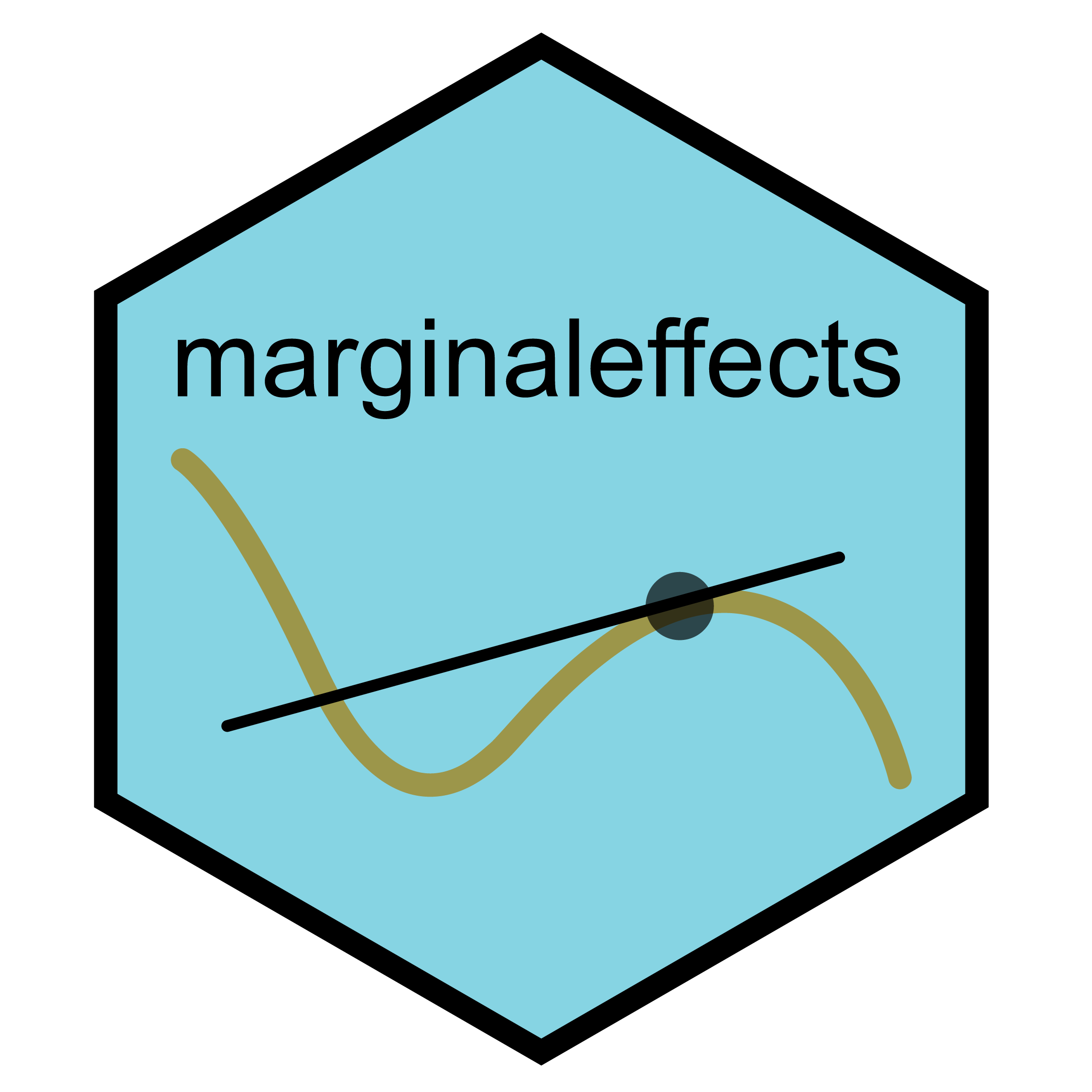# The `marginaleffects` package for `R`Compute and plot predictions, slopes, marginal means, and comparisons (contrasts, risk ratios, odds ratios, etc.) for over 70 classes of statistical models in R. Conduct linear and non-linear hypothesis tests, as well as equivalence tests using the delta method.

The website includes a “Get started” tutorial and over 25 vignettes, case studies, and technical notes.

# Installation

Install the latest CRAN release:

``install.packages("marginaleffects")``

Install the development version:

``````install.packages(
c("marginaleffects", "insight"),
repos = c("https://vincentarelbundock.r-universe.dev", "https://easystats.r-universe.dev"))``````

Restart `R` completely before moving on.

# Why?

Parameter estimates are often hard to interpret substantively, especially when they are generated by complex models with non-linear components or transformations. Many applied researchers would rather focus on simple quantities of interest, which have straightforward scientific interpretations. Unfortunately, these estimands (and their standard errors) are tedious to compute. Moreover, the different modeling packages in `R` often produce inconsistent objects that require special treatment.

`marginaleffects` offers a single point of entry to easily interpret the results of over 73 classes of models, using a simple and consistent user interface.

Benefits of `marginaleffects` include:

• Powerful: It can compute predictions, comparisons (contrasts, risk ratios, etc.), slopes, and conduct hypothesis tests for 73 different classes of models in `R`.
• Simple: All functions share a simple and unified interface.
• Documented: Each function is thoroughly documented with abundant examples. The website includes 20,000+ words of vignettes and case studies.
• Efficient: Some operations are orders of magnitude faster than with the `margins` package, and the memory footprint is much smaller.
• Valid: When possible, numerical results are checked against alternative software like `Stata` or other `R` packages.
• Thin: Few dependencies.
• Standards-compliant: `marginaleffects` follows “tidy” principles and returns objects that work with standard functions like `plot`, `summary()`, `tidy()`, and `glance()`. These objects are easy to program with and feed to other packages like `modelsummary`.
• Extensible: Adding support for new models is very easy, often requiring less than 10 lines of new code. Please submit feature requests on Github.
• Active development: Bugs are fixed promptly.

# What?

The `marginaleffects` package allows `R` users to compute and plot three principal quantities of interest: (1) predictions, (2) comparisons, and (3) slopes. In addition, the package includes a convenience function to compute a fourth estimand, “marginal means”, which is a special case of averaged predictions. `marginaleffects` can also average (or “marginalize”) unit-level (or “conditional”) estimates of all those quantities, and conduct hypothesis tests on them.

Predictions:

The outcome predicted by a fitted model on a specified scale for a given combination of values of the predictor variables, such as their observed values, their means, or factor levels. a.k.a. Fitted values, adjusted predictions. `predictions()`, `avg_predictions()`, `plot_predictions()`.

Comparisons:

Compare the predictions made by a model for different regressor values (e.g., college graduates vs. others): contrasts, differences, risk ratios, odds, etc. `comparisons()`, `avg_comparisons()`, `plot_comparisons()`.

Slopes:

Partial derivative of the regression equation with respect to a regressor of interest. a.k.a. Marginal effects, trends. `slopes()`, `avg_slopes()`, `plot_slopes()`.

Marginal Means:

Predictions of a model, averaged across a “reference grid” of categorical predictors. `marginalmeans()`.

Goal

Function

Predictions

`predictions()`

`avg_predictions()`

`plot_predictions()`

Comparisons

`comparisons()`

`avg_comparisons()`

`plot_comparisons()`

Slopes

`slopes()`

`avg_slopes()`

`plot_slopes()`

Marginal Means

`marginal_means()`

Grids

`datagrid()`

`datagridcf()`

Hypothesis & Equivalence

`hypotheses()`

Bayes, Bootstrap, Simulation

`posterior_draws()`

`inferences()`